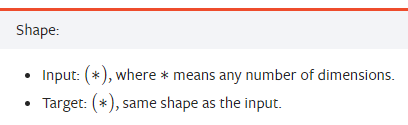# TypeError: empty(): argument 'size' must be tuple of ints, but found element of type torch.Size at pos 2

Hi i need help regrading this issues TypeError: empty(): argument ‘size’ must be tuple of ints, but found element of type torch.Size at pos 2

Can you share the code snippet, you are passing torch.Size to empty whereas it expects tuple. If you are trying to create an empty tensor with certain dimensioned tensor x. Call torch.empty(x.shape) instead of torch.empty(x.size()).

`Variable()` has been deprecated.

You can simply set the flag `requires_grad` to `True` when creating a tensor.

``````h_0 = torch.zeros(self.num_layers, x.size(0), self.hidden_size, requires_grad=True)
c_0 = torch.zeros(self.num_layers, x.size(0), self.hidden_size, requires_grad=True)
``````

its not working still got same error

It would really help if you posted where the error is occurring. You could, for example, post the error stack trace or a reproducible code to get this error.

As @Sairam954 has already said, it appears that you are trying to create an empty tensor by passing a tuple and he has given some solutions. Another solution would be to use `empt_like` (docs).

However, in the code that you posted there is no empty tensor created.

``````
* for epoch in range(num_epochs):
outputs = lstm1.forward(X_train_tensors_final) #forward pass

# obtain the loss function
loss = criterion(outputs, X_test_tensors_final)

loss.backward() #calculates the loss of the loss function

optimizer.step() #improve from loss, i.e backprop
if epoch % 100 == 0:
print("Epoch: %d, loss: %1.5f" % (epoch, loss.item()))
[details="Summary"]
This text will be hidden
[/details]

``````

*RuntimeError: The size of tensor a (6) must match the size of tensor b (9) at non-singleton dimension 2

@Matias_Vasquez

Hi,

can you print the entire stacktrace?

This way it will be easier to determine where the error is happening and why.

``````/usr/local/lib/python3.7/dist-packages/torch/nn/modules/loss.py:529: UserWarning: Using a target size (torch.Size([128, 2947, 9])) that is different to the input size (torch.Size([384, 6])). This will likely lead to incorrect results due to broadcasting. Please ensure they have the same size.
return F.mse_loss(input, target, reduction=self.reduction)
---------------------------------------------------------------------------
RuntimeError                              Traceback (most recent call last)
<ipython-input-16-d3c6c29eeb10> in <module>()
4
5   # obtain the loss function
----> 6   loss = criterion(outputs, X_test_tensors_final)
7
8   loss.backward() #calculates the loss of the loss function

3 frames
73     if has_torch_function(tensors):
Here is the documentation for `MSELoss`. As you can see on the bottom, it says that the `input` shape and `target` shape have to be the same.It seems that you are using the output of your model as the `input` for your criterion (this is correct, the prediction should go here). However, the `target` is `X_test_tensors_final`. This should be the ground truth labels to the input data you gave your model to classify.